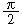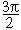home | O'Reilly's CD bookshelfs | FreeBSD | Linux | Cisco | Cisco Exam2.12. Calculating More Trigonometric Functions

Problem

You want to calculate values for trigonometric functions like sine, tangent, or arc-cosine.

Solution

Perl provides only sin , cos , and atan2 as standard functions. From these, you can derive tan and the other trig functions:

sub tan {
my \$theta = shift;

return sin(\$theta)/cos(\$theta);
}

The POSIX module provides a wider range of trig functions:

use POSIX;

\$y = acos(3.7);

The Math::Trig module provides a complete set of functions and supports operations on or resulting in complex numbers:

use Math::Trig;

\$y = acos(3.7);

Discussion

The tan function will cause a division-by-zero exception when \$theta is,, and so on, because the cosine is 0 for these values. Similarly, tan and many other functions from Math::Trig may generate the same error. To trap these, use eval :

eval {
\$y = tan(\$pi/2);
} or return undef;2.11. Doing Trigonometry in Degrees, not Radians2.13. Taking Logarithms